Disclosure: This post may contain affiliate links, meaning when you click the links and make a purchase, we receive a commission.

# How to Sum Multiple Rows and Columns in Excel

Summing up multiple rows and column in Excel is important for many purposes. In this article, we will see different techniques on how to sum multiple rows and columns in Excel. Functions like SUM, SUMPRODRUCT will be used here in this article along with feature like Autosum.

## How to perform AutoSum in Excel

Let`s say we have a worksheet which contains the monthly sales of different electrical appliances. Now, you wish to calculate the total monthly sales of all the products. You can easily do that by using the AutoSum feature. The procedure of performing the AutoSum feature is given below.

• First, select the cell where you want to find the total sales amount. In our case, we want to find the totals sales of January in cell B10. In the Formulas tab under the Editing option, click on to the AutoSum option and there click on the SUM option.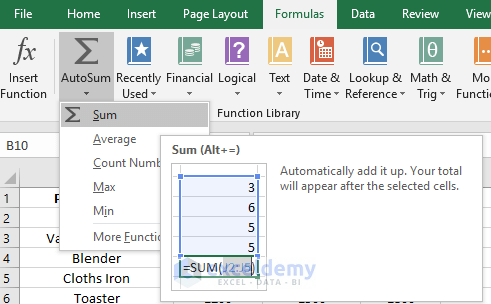• Now after selecting the SUM option you will see the SUM function appears in cell B10 along with the range.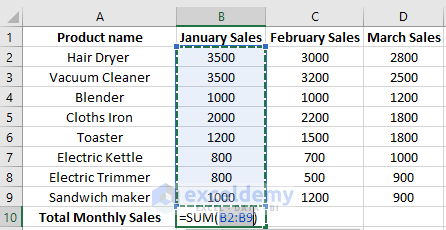• Now press Enter to perform this AutoSum feature.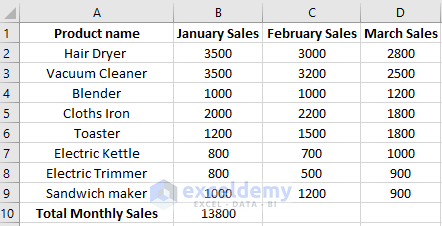• After this, drag this formulated cell in the right side in cell C10 and D10 to find the total sales amount of the month of February and March.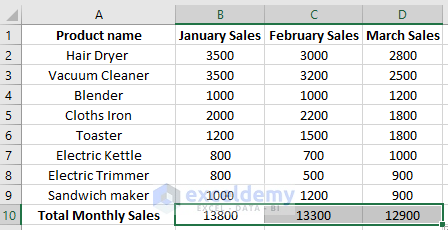Note: Instead of using the Autosum feature from Formulas tab, you can also use the keyboard shortcut “Alt+=”.

## Using the SUM/SUMPRODUCT Function for Multiple Columns

Instead of using the AutoSum feature you can also use the SUM function directly to calculate the total sales for a month. For this type =SUM(B2:B9).Now after pressing Enter, drag this formulated cell in cell C10 and D10 to calculate the total sales for the month of February and March.Instead of SUM function, you can also use the SUMPRODUCT function to perform this calculation.

## Using the SUM/SUMPRODUCT Function for Multiple Rows

Now let`s say you want to find out the total sales of the specific product. In this case, we will be using the same formula but the calculation will be done in row-wise.

• To calculate the total sales amount of hair dryer, type the formula =SUM(B2: D2) in cell E2.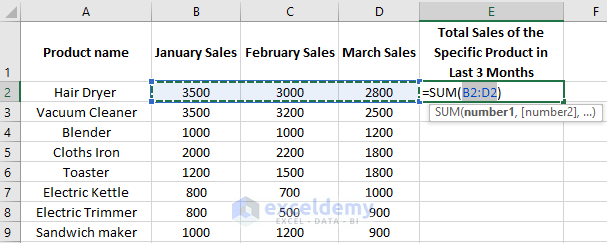• Now press Enter to see the result.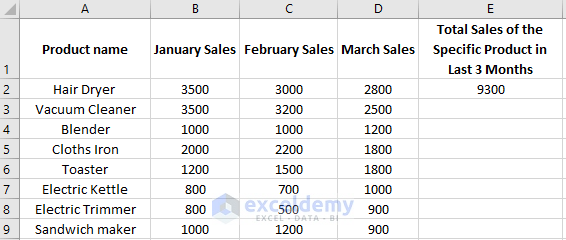• Now drag down the formulated cell downwards or double-click on the Fill handle to find out the totals sales of the other products.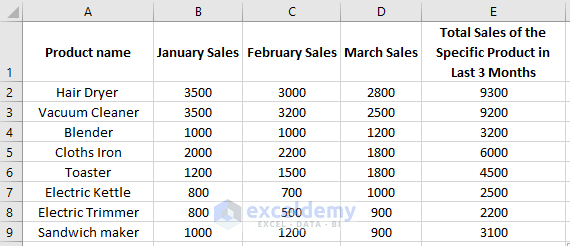## Finding Total by Defining Columns Range Instead of Cells Range

You can add multiple columns by specifying the columns name instead of cells range. Let`s say we want to find out the total sales of the products for the last 3 months. Here the months are defined in sales B, C and D. Type the formula =SUM(\$B:\$D) in cell F11. This will sum up all the values of columns B, C and D. The usefulness of using this formula is that, whenever you will place new products name along with the sales value, it will get updated automatically if the new values are in this column range.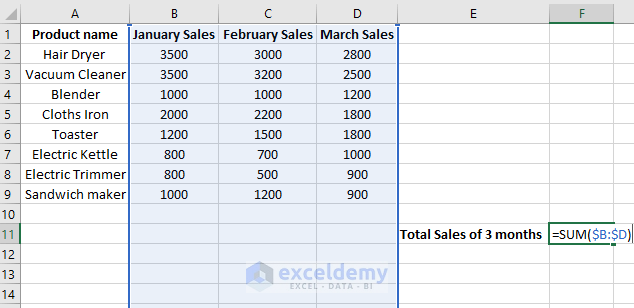After pressing Enter you will get the below result.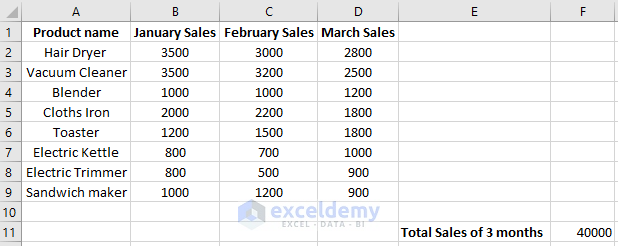Note: If you place this formula in the defined column range of the formula which is \$B:\$D. The formula will not work as it creates a circular cell reference. It will give you the result “0”.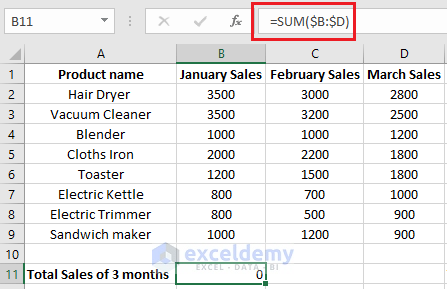## Finding Total by Defining Rows Range Instead of Cells Range

In the same way, you can find out the total sales of individual products by specifying the row range instead of cell range in the formula. Let`s say you want to find the total sales of Hair Dryer, Blender, and Toaster. For calculating the total sales of Hair Dryer you can use the formula, =SUM(2:2). In the same way, the total sales of other products can be found.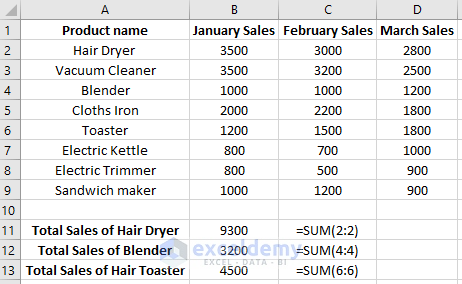Note: Like before you can’t use this formula in the defined row range of the formula as it will create the circular cell reference. If you use this formula in the same row it will give a “0” result.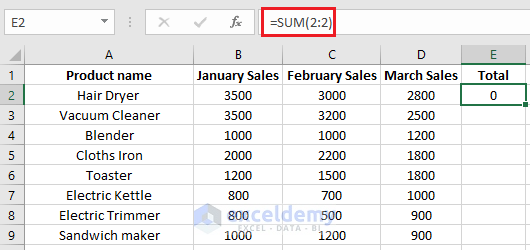## Summing Data in a Table using Total Row in Excel

Suppose the example that we are working on is now defined as a table in the worksheet. When you have a data table in your worksheet you can insert the Total Row option for summing up the data in a table. For inserting the Total Row, first, select any cell of the table and in the Design tab, select the Total Row under the Table Style option.You will see the sum value of column D after selecting the Total Row option. It means the total sales for March will be seen with this.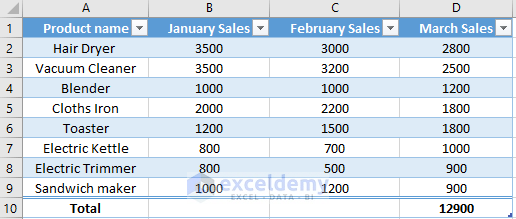The most interesting part of this total Row option is that you will get the drop-down list option for additional calculation at the end of your table. So, from this, you can easily find the total sales of month January and February. Select the cell B10 and you will see the drop-down list. From there select the SUM option. The sum value of January sales will be found from this.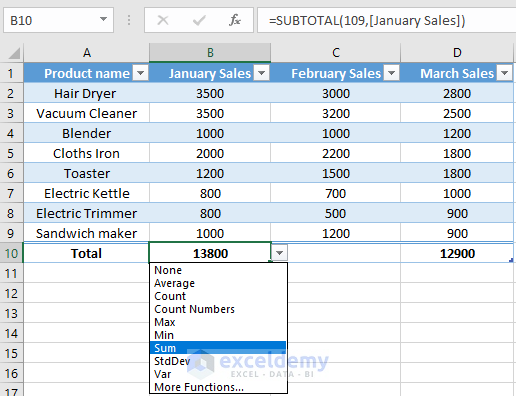Now perform this for cell C10 also. From this all the sum values of column B, C and D will be found.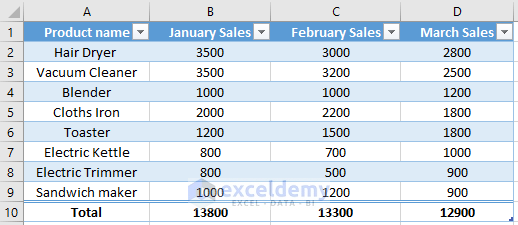How to Sum Multiple Rows and Columns in Excel